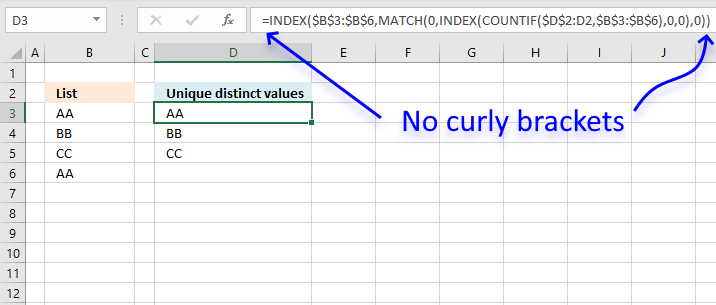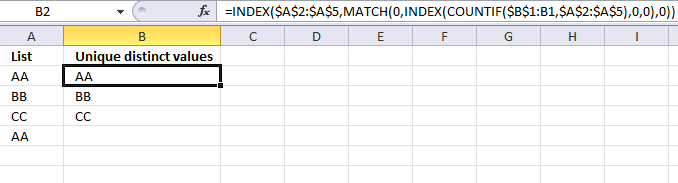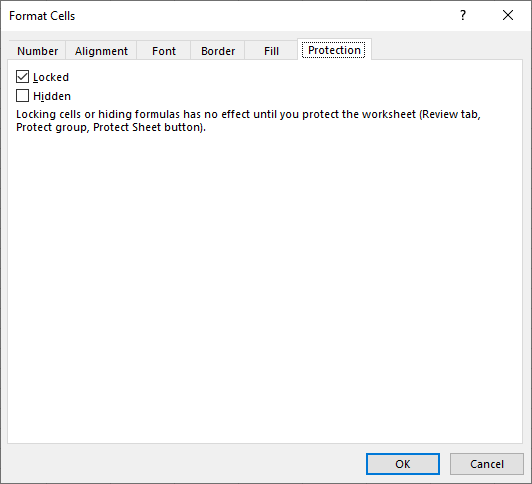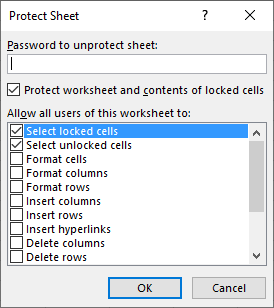Author: Oscar Cronquist Article last updated on December 18, 2019This article explains how to avoid array formulas if needed. It won't work with all array formulas, however, smaller ones seem fine.

Why would you want to avoid array formulas? An unexperienced Excel user may turn it into a regular formula by editing it and then press Enter. That will break the array formula, and often but not always, an error will be returned.

Now, if you can't avoid an array formula, you could lock the cell containing the array formula and protect the worksheet. I will explain how to do this, as well.

Kamran Mumtaz askedIs there any way to use VLOOKUP for multiple criteria and I do not want to use CSE?;

CSE is an abbreviation for CTRL + SHIFT + ENTER, which is how you enter an array formula.

My answer: No, not to my knowledge.

Kamran Mumtaz:  This is the formula given by Aladin Akyurek without (CSE)...

=INDEX(Sheet3!\$B\$2:\$B\$65, MATCH(1,INDEX((Sheet3!\$C\$2:\$C\$65=E\$1)*(Sheet3!\$A\$2:\$A\$65=\$A3), 0, 1), 0))

This formula is exciting, I have never seen this approach before. It looks like an array formula, but it is not. It opens up possibilities that I have not thought about before.

### Example 1,This formula derives from the following article: Extract a unique distinct list

The original array formula:

=INDEX(\$A\$2:\$A\$5,MATCH(0,COUNTIF(\$B\$1:B1,\$A\$2:\$A\$5),0))

The new regular formula in cell B2:

=INDEX(\$A\$2:\$A\$5,MATCH(0,INDEX(COUNTIF(\$B\$1:B1,\$A\$2:\$A\$5),0,0),0))

The new formula is NOT an array formula! Amazing! I am not sure if all array formulas can be converted to regular formulas, but some can.

Update: I am now using an even smaller regular formula that is not using this method at all. The LOOKUP function can do things that would generally require an array formula. See this article for more: Extract a unique distinct list

### Explaining formula in cell B2

The formula in column B extracts unique distinct values from column A without the need to create an array formula.

I will explain below the calculation steps and the workaround that makes this possible.

#### Step 1 - Count cells based on condition(s)

The COUNTIF function counts cells in a cell range based on a condition. COUNTIF(rangecriteria), range is \$B\$1:B1 and criteria is \$A\$2:\$A\$5.

This seems perhaps wrong, but it is not. \$ B\$1:B1 is a cell reference that expands when the cell is copied and pasted to cells below. The dollar signs in \$B\$1 make this part of the cell ref absolute, meaning it is locked.

The other part is B1, and that cell ref is relative, meaning it changes from cell to cell, for this to work you need to copy the cell and not the formula.

COUNTIF(\$B\$1:B1,\$A\$2:\$A\$5)

becomes

COUNTIF("Unique distinct values", {"AA";"BB";"CC";"AA"})

and returns {0; 0; 0; 0}.

This means that "Unique distinct values" is not equal to any value in this array {"AA";"BB";"CC";"AA"}. This method requires you usually to enter the formula as an array formula. However, the next step is a workaround.

#### Step 2 - Create a regular formula

Generally, If the COUNTIF function returns an array, this would require you to enter the formula as an array formula. However, if you encapsulate the COUNTIF function with the INDEX function you can create a workaround. Unfortunately it comes with a cost, the formula grows larger.

INDEX(COUNTIF(\$B\$1:B1,\$A\$2:\$A\$5),0,0)

becomes

INDEX({0; 0; 0; 0},0,0)

and returns {0; 0; 0; 0}.

You can shorten the INDEX function to

INDEX(COUNTIF(\$B\$1:B1,\$A\$2:\$A\$5),)

and the result will be the same.

#### Step 3 - Find first value not displayed

The MATCH function returns the relative position of the first value that is equal to 0 (zero). MATCH(lookup_value, lookup_array, [match_type])

MATCH(0,INDEX(COUNTIF(\$B\$1:B1,\$A\$2:\$A\$5),0,0),0)

becomes

MATCH(0,{0; 0; 0; 0},0)

and returns 1. 0 (zero) is equal to the first value in the array.

#### Step 4 - Return value based on row number

The INDEX function returns a value from a given cell range based on a specified row number. INDEX(array[row_num][column_num])

INDEX(\$A\$2:\$A\$5, MATCH(0, INDEX(COUNTIF(\$B\$1:B1, \$A\$2:\$A\$5), 0, 0), 0))

becomes

INDEX(\$A\$2:\$A\$5, 1)

and returns "AA" in cell B2.

### Example 2,This example comes from this article: How to return multiple values using vlookup. The formula returns values based ona condition, if value in cell E1 is equal to one or many values in cell range A2:A5 the formula will return the corresponding values from B2:B5.

Original array formula:

=INDEX(\$B\$2:\$B\$5, SMALL(IF(\$E\$1=\$A\$2:\$A\$5, MATCH(ROW(\$B\$2:\$B\$5),ROW(\$B\$2:\$B\$5)), ""), ROW(A1)))

The new regular formula in cell E2:

=INDEX(\$B\$2:\$B\$5, SMALL(INDEX((\$A\$2:\$A\$5=\$E\$1)*(MATCH(ROW(\$B\$2:\$B\$5), ROW(\$B\$2:\$B\$5)))+(\$A\$2:\$A\$5<>\$E\$1)*1048577, 0, 0), ROW(A1)))

### Explaining formula in cell E2

#### Step 1 - Compare values

The equal sign lets you check if a value is equal to another value or multiple values.

\$A\$2:\$A\$5=\$E\$1

becomes

{"AA";"BB";"CC";"AA"}="AA"

and returns {TRUE; FALSE; FALSE; TRUE}.

#### Step 2 - Create an array of numbers from 1 to n

We need an array of numbers starting from 1 to n where n is the total number of rows in the cell range.

MATCH(ROW(\$B\$2:\$B\$5), ROW(\$B\$2:\$B\$5))

becomes

MATCH({2;3;4;5}, {2;3;4;5})

and returns {1;2;3;4}. There are four rows in cell range \$B\$2:\$B\$5.

#### Step 3 - Multiply logical expression with array

The asterisk character allows you to multiply numbers, arrays, and constants in a formula. Use parentheses to control the order of operation.

(\$A\$2:\$A\$5=\$E\$1)*(MATCH(ROW(\$B\$2:\$B\$5), ROW(\$B\$2:\$B\$5)))

becomes

{TRUE; FALSE; FALSE; TRUE}*(MATCH(ROW(\$B\$2:\$B\$5), ROW(\$B\$2:\$B\$5)))

becomes

{TRUE; FALSE; FALSE; TRUE}*{1;2;3;4}

and returns {1;0;0;4}.

TRUE and FALSE are boolean values, their numerical equivalents are 1 and 0 (zero). For example TRUE*1=1, FALSE*1=0.

#### Step 4 - Check if values are not equal to value in E1

This step  contains a logical expression that returns TRUE if a value is not equal to cell E1 and FALSE if equal. Combine a less than and greater than character to check if values are not equal.

\$A\$2:\$A\$5<>\$E\$1

becomes

{"AA";"BB";"CC";"AA"}<>"AA"

and returns

{FALSE; TRUE; TRUE; FALSE}.

#### Step 5 - Multiply with a value greater than the maximum row number

A worksheet can contain up to 1048576 rows, a larger number will return an error in step 9.

(\$A\$2:\$A\$5<>\$E\$1)*1048577

becomes

{FALSE; TRUE; TRUE; FALSE}*1048577

and returns

{0; 1048577; 1048577; 0}

#### Step 6 - Add arrays

The plus sign + lets you add arrays.

(\$A\$2:\$A\$5=\$E\$1)*(MATCH(ROW(\$B\$2:\$B\$5), ROW(\$B\$2:\$B\$5)))+(\$A\$2:\$A\$5<>\$E\$1)*1048577

becomes

{1;0;0;4}+(\$A\$2:\$A\$5<>\$E\$1)*1048577

becomes

{1;0;0;4}+{0; 1048577; 1048577; 0}

and returns

{1; 1048577; 1048577; 4}

#### Step 7 - Remove need for array formula

The INDEX function lets you do calculations without requiring you to create an array formula.

INDEX((\$A\$2:\$A\$5=\$E\$1)*(MATCH(ROW(\$B\$2:\$B\$5), ROW(\$B\$2:\$B\$5)))+(\$A\$2:\$A\$5<>\$E\$1)*1048577, 0, 0)

becomes

INDEX({1; 1048577; 1048577; 4}, 0, 0)

and returns

{1; 1048577; 1048577; 4}

#### Step 8 - Extract k-th smallest number in array

The SMALL function extracts the k-th smallest number in the array, this step makes sure that different values display in every cell.

SMALL(INDEX((\$A\$2:\$A\$5=\$E\$1)*(MATCH(ROW(\$B\$2:\$B\$5), ROW(\$B\$2:\$B\$5)))+(\$A\$2:\$A\$5<>\$E\$1)*1048577, 0, 0), ROW(A1))

becomes

SMALL({1; 1048577; 1048577; 4}, ROW(A1))

The ROW function contains a relative cell reference that changes when you copy the cell and paste to cells below.

SMALL({1; 1048577; 1048577; 4}, ROW(A1))

becomes

SMALL({1; 1048577; 1048577; 4}, 1)

and returns 1.

#### Step 9 - Return value from cell range

This step utilizes the INDEX function to fetch a value from cell range B2:B5.

INDEX(\$B\$2:\$B\$5, SMALL(INDEX((\$A\$2:\$A\$5=\$E\$1)*(MATCH(ROW(\$B\$2:\$B\$5), ROW(\$B\$2:\$B\$5)))+(\$A\$2:\$A\$5<>\$E\$1)*1048577, 0, 0), ROW(A1)))

becomes

INDEX(\$B\$2:\$B\$5, 1)

and returns 1 in cell E2.

The IF function has a logical expression that compares multiple values to a condition.

### Get the Excel fileno-arrays.xlsx

### How to lock cells

You can lock cells so they can't be edited and that would make it safer to use array formulas. Keep in mind that there are tools out there that can open up password-protected sheets easily.

All cells in a worksheet are locked by default, you can verify this. Select any cell in your worksheet, press CTRL + 1 to format cell. Go to tab "Protection".This tab shows you if the cell is locked, the checkbox is enabled if it is locked. To lock cells you need to protect the worksheet.

1. Go to the worksheet you want to protect.
2. Go to tab "Review" on the ribbon.
3. Press with mouse on "Protect sheet" button.4. Enter a password you want to use. Remember it because you will need it next time you want to unprotect the worksheet.
5. Press with left mouse button on OK button.

What do you prefer? Array formulas or somewhat longer and more complicated regular formulas?